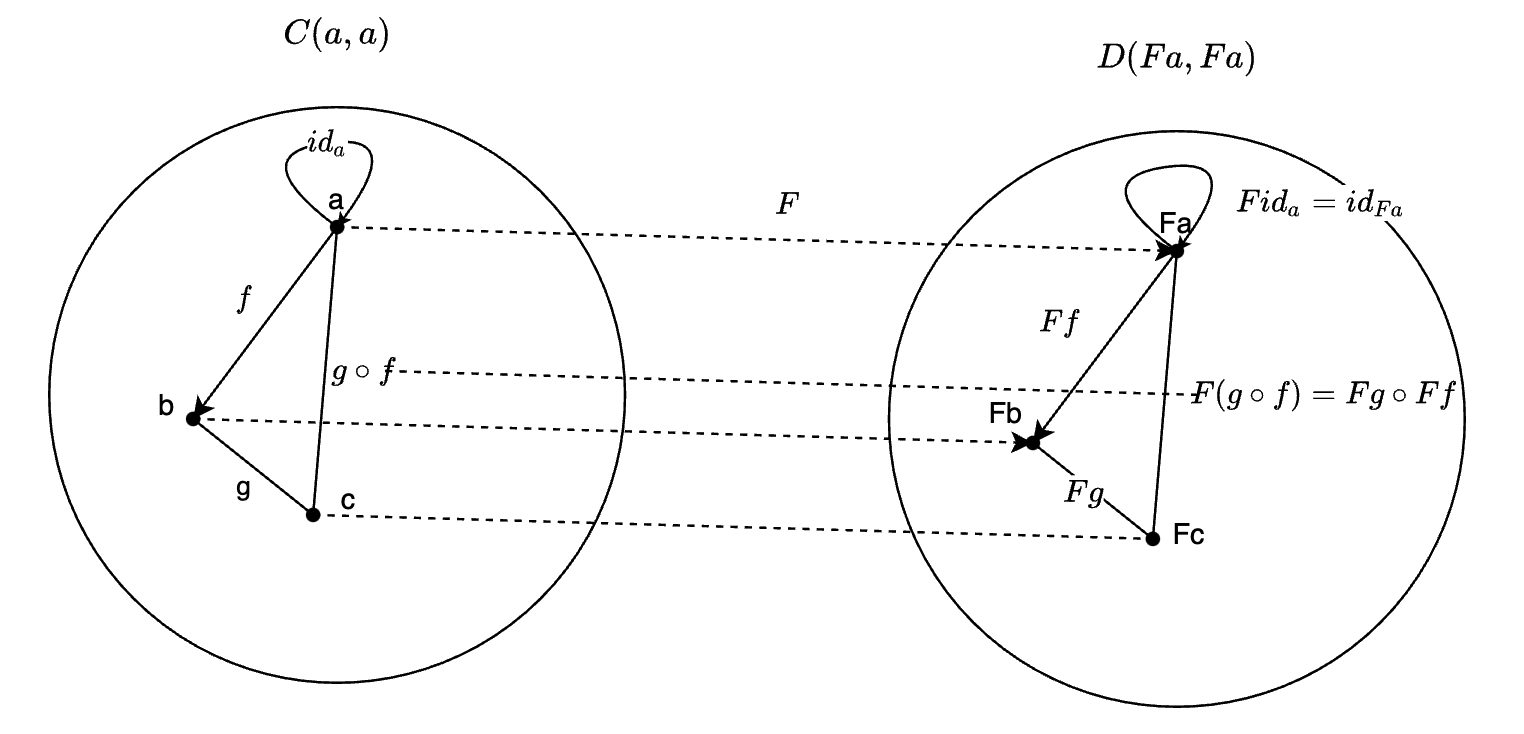### JavaScript中的map方法(本质上)是什么？⌗

const array1 = [1, 2, 3]
const array2 = array1.map(i => i + 1)
const array3 = array1.map(i => String.fromCharCode(96 + i))


array2是[2, 3, 4]，array3是['a', 'b', 'c']

array是一个容器，i => i + 1这个方法无法直接作用于array上，通过map方法，将方法作用于array容器中的元素上，并将结果重新放回容器中

const map = (func, array) => array.map((v) => func(v));


map :: (A -> B) -> T[A] -> T[B]

### Functor的定义⌗Functor本质上是将一个Category映射到另一个Category，并保持Category的结构不变。如果源Category和目标Category是同一个，那这个Functor叫Endofunctor

### 基于Functor重新理解map⌗

function curry(func1, arg1) {
return (arg2) => func1(arg1, arg2);
}

function compose(func1, func2) {
return (arg) => func2(func1(arg))
}


const arr = [1, 2, 3]

// 单个参数的函数
const add1 = (x) => x + 1;

// ret1 = [2, 3, 4]

map(console.log, ret1); // 2 3 4

// 基于Functor的定义，可知道：
// map(f . g) = map(f) . map(g)

// 第2个函数
const multiple2 = (x) => x * 2;

// 这里相当于执行 map(f . g)
map(console.log, ret2); // 4 6 8

// 将multiple2映射到array空间去
const arrayMultiple2 = curry(map, multiple2);

// 这里相当于 map(f) . map(g)